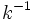# Commutator of two subgroups

## Definition

### Symbol-free definition

The commutator of two subgroups of a group is defined as the subgroup generated by commutators between elements in the two subgroups.

### Definition with symbols

Suppose$G$ is a group and$H$ and$K$ are subgroups of$G$. The commutator of the subgroups$H$ and$K$, denoted$[H,K]$, is defined as:$[H,K] := \langle [h,k] \mid h \in H, k \in K \rangle$

where:$[h,k] = h^{-1}k^{-1}hk$

is the commutator of the elements$h$ and$k$.

Note that there are two conventions for commutators; in some other conventions:$[h,k] = hkh^{-1}k^{-1}$.

Whatever the convention, the set of commutators is the same; the commutator of$h$ and$k$ in the former convention equals the commutator of$h^{-1}$ and$k^{-1}$ in the latter convention.

## Facts

### Commutator, closure and join

If$H, K \le G$ are subgroups, let$H^K$ denote the closure of$H$ under the action of$K$. Define$K^H$ analogously. We then have:

•$[H,K]$ is a normal subgroup inside$H^K$. In fact,$H^K = H[H,K]$, where$H$ normalizes$[H,K]$.
•$[H,K]$ is a normal subgroup inside$K^H$. In fact,$K^H = K[H,K]$ where$K$ normalizes$[H,K]$.
•$[H,K]$ is a normal subgroup inside$\langle H, K \rangle$. Both$H^K$ and$K^H$ are normal inside$\langle H, K \rangle$, with$\langle H, K \rangle = KH^K = HK^H$.

For full proof, refer: Commutator of two subgroups is normal in join

### Normalizing characterized in terms of commutators

For subgroups$H,K \le G$,$K$ is contained in the normalizer of$H$ if and only if$[H,K] \le H$. (In particular,$H$ is normal if and only if$[H,G] \le H$).

Similarly,$H$ is contained in the normalizer of$K$ if and only if$[H,K] \le K$. Thus, the subgroups$H$ and$K$ normalize each other iff$[H,K] \le H \cap K$. In particular, if both subgroups are normal, their commutator is contained in their intersection.

### Permuting subgroups characterized in terms of commutators

Subgroups$H, K \le G$ are permuting subgroups if and only if$[H,K] \le HK$; in other words, the commutator of the subgroups is contained in their product.

### Normal closure and quotient

The commutator of two subgroups need not, in general, be a normal subgroup. The normal closure of the commutator of two subgroups is of greater interest. If$L$ denotes the normal closure of$[H,K]$ for$H,K$ subgroups of$G$, then the images of$H$ and$K$ in$G/L$ commute element-wise. Conversely, any normal subgroup for which the images of$H$ and$K$ commute element-wise in the quotient, must be contained in$L$.

However, in the special case when both$H$ and$K$ are normal, the commutator of the subgroups is also normal. Further information: Commutator of normal subgroups is normal